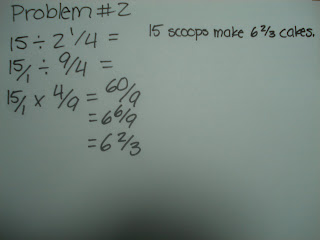### Joseph's Last Fraction Post

Sunday, May 10, 2009

1) Find the common denomintor

2) Add numerator to numerator and denominator to denominator

3) Simplify if possible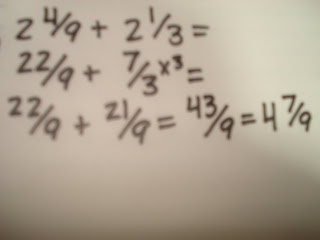1) Convert mixed numbers into improper fractions

2) Find a common denominator

3) Add numerator to numerator and denominator to denominator

4) Convert answer into mixed number

5) Simplify if possible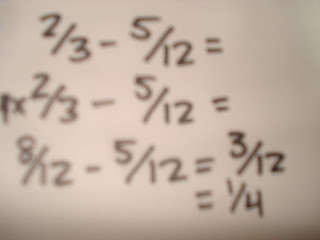SUBTRACTING FRACTIONS:

1) Find the common denominator

2) Subtract numerator from numerator and denominator from denominator

3) Simplify if possibleMULTIPLYING MIXED NUMBERS:

1) Convert any mixed numbers into improper fractions

2) Multiply numerator with numerator and denominator with denominator

3) Convert answer into mixed number

4) Simplify if possible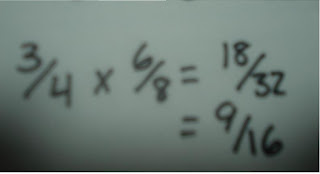MULTIPLYING FRACTIONS:

1) Multiply numerator with numerator and denominator with denominator

2) Simplify if possible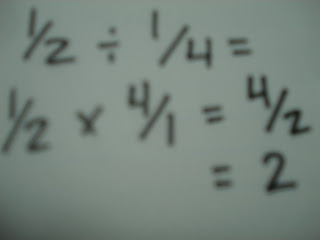DIVIDING FRACTIONS:

1) Find the reciprocal ( flip one of the fractions upside down)

2) Multiply numerator with numerator and denominator with denominator

3) Simplify if possible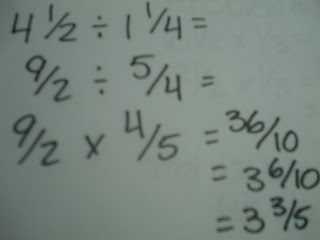DIVIDING MIXED NUMBERS:

1) Convert mixed numbers into improper fractions

2) Find the reciprocal ( flip one of the fractions upside down)

4) Multiply numerator with numerator and denominator with denominator

5) Convert answer into mixed number

6) Simplify if possible